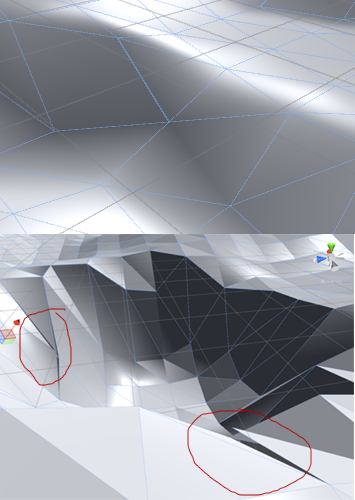# Problem with setting vertices positions and smoothing groups.

So, I have a two-part problem. I’ve started playing around with mesh/vertex manipulation in code with the information available in the docs. I have a planar that, when hit with a raycast takes the closest x amount of vertices from the hit point and lowers their Y position by 0.02. This works fine.
I wanted to keep the hard, visible edges, so I exported a plane (both flat and curved, to test both) from 3DS with all smoothing groups cleared. With the flat plane, it works fine, but automatically smooths all of the normals, which I don’t want. With the curved plane, it smooths some of the normals, but also breaks some of the edges.
I’ve made sure there are no overlapping vertices, tried messing with the normals and tangents settings in the importer dialog but nothing seems to be working so far.
Does anyone know how to stop both/either of these from happening?

``````#pragma strict

private var distances : float[];
private var allVerts : Vector3[];
private var indexNum : int[];

var theMesh : Mesh;
var countLength : int;

function Start(){
theMesh = this.GetComponent.<MeshFilter>().mesh;
}

function CheckVerts(hitPos : Vector3){
indexNum = new int[countLength];
var targetPosition : Vector3 = transform.TransformPoint(hitPos);
allVerts = theMesh.vertices;
distances = new float[allVerts.Length];
for(var xx : int = 0; xx < allVerts.Length; xx++){
var myPosition : Vector3 = transform.TransformPoint(allVerts[xx]);
distances[xx] = Vector3.Distance(targetPosition, myPosition);
}
for(var yy : int = 0; yy < indexNum.Length; yy++){
var currentLowestIndex : int;
var lowestNum : float = 1000;
for(var zz : int = 0; zz < distances.Length; zz++){
if(distances[zz] >= 0 && distances[zz] < lowestNum){
currentLowestIndex = zz;
lowestNum = distances[zz];
}
}
indexNum[yy] = currentLowestIndex;
distances[currentLowestIndex] = -100;
}
ChangePositions();
}

function ChangePositions(){
for(var xx : int = 0; xx < indexNum.Length; xx++){
allVerts[indexNum[xx]] = Vector3(allVerts[indexNum[xx]].x, allVerts[indexNum[xx]].y - 0.02, allVerts[indexNum[xx]].z);
}
theMesh.vertices = allVerts;
theMesh.RecalculateBounds();
theMesh.RecalculateNormals();
this.GetComponent.<MeshCollider>().sharedMesh = theMesh;
}
``````So I’ve managed to sort the problem with the normal smoothing out, but the edges are still breaking.

edit(moved from comment)

So I’ve solved the second problem as well. According to a really old thread that was difficult to find, having a non-smoothed object uses multiple vertices to render additional normal directions, so the edges weren’t ‘breaking’ per-se, the vertices simply weren’t all being moved.
I’ve written something to get all the duplicate verts here, if anyone’s interested.
This is quite an expensive operation, however, so any refinement or less CPU-heavy code would be greatly appreciated!

``````function CheckVerts(hitPos : Vector3){
var targetPosition : Vector3 = hitPos;;
distances = new float[allVerts.Length];
for(var xx : int = 0; xx < allVerts.Length; xx++){
var myPosition : Vector3 = transform.TransformPoint(allVerts[xx]);
distances[xx] = Vector3.Distance(targetPosition, myPosition);
}
for(var yy : int = 0; yy < indexNum.Length; yy++){
var currentLowestIndex : int;
var lowestNum : float = 1000;
for(var zz : int = 0; zz < distances.Length; zz++){
if(distances[zz] >= 0 && distances[zz] < lowestNum){
currentLowestIndex = zz;
lowestNum = distances[zz];
}
}
indexNum[yy] = currentLowestIndex;
distances[currentLowestIndex] = -100;
}
targetPosition = Vector3.zero;
GetDuplicates();
}

function GetDuplicates(){
var tempArr = new Array();
for(var xx : int = 0; xx < indexNum.Length; xx++){
for(var zz : int = 0; zz < allVerts.Length; zz++){
if(allVerts[zz] == allVerts[indexNum[xx]]){
tempArr.Push(zz);
}
}
}
var dups : int[] = tempArr.ToBuiltin(int) as int[];
allPoints = dups;
tempArr = null;
dups = null;
}
``````

Basically, this gets the X amount of vertices closest to the hit point and stores the index numbers of those vertices in an array. It then takes that array and iterates through it, checking the rest of the verts for identical positions, which are added to a JS array. It converts the JS array to a built-in, which can then be modifed however you wish.